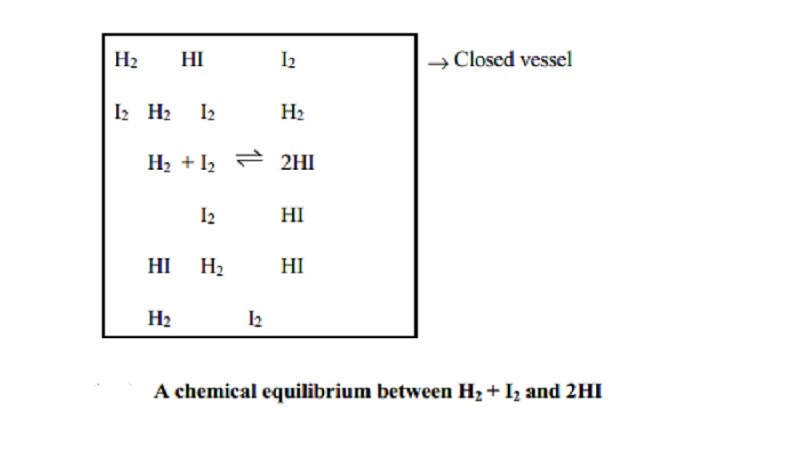Home | | Chemistry | | Chemistry | Dynamic Equilibrium: Characteristics, Equilibrium in physical and chemical processes

# Dynamic Equilibrium: Characteristics, Equilibrium in physical and chemical processesWhen a reversible reaction attains equilibrium it appears that the concentrations of individual reactants and that of the products remain constant with time. Apparently, the equilibrium appears as dead (or) as not proceeding.

Dynamic Equilibrium

When a reversible reaction attains equilibrium it appears that the concentrations of individual reactants and that of the products remain constant with time. Apparently, the equilibrium appears as dead (or) as not proceeding. Actually, the reactant molecules are always reacting to form the product molecules. When the product molecules are able to react with themselves under the same experimental condition to form the same amount of reactants simultaneously (at the same time) in an equal rate of the forward reaction, then the process is a ceaseless phenomenon. Thus chemical equilibrium is dynamic when the forward and reverse reactions take place endlessly and simultaneously with equal rates. Therefore chemical equilibrium is called as dynamic equilibrium.

Characteristics of Chemical Equilibrium

(i)  Constancy of concentrations

When a chemical equilibrium is established in a closed vessel at constant temperature, the concentrations of various species like reactants and products remain unchanged.

The reaction mixture consisting of reactants and products at equilibrium is called as equilibrium mixture.

The concentrations of reactants and products at equilibrium are called as equilibrium concentrations.

(ii) Equilibrium can be initiated from either side. The state of equilibrium of a reversible reaction can be arrived at whether we start from reactants or products.

For example, this equilibrium H2(g) + I2(g) _ 2HI(g) can be achieved whether we start with H2 and I2 or with HI.

(iii) Equilibrium cannot be attained in an open vessel

Only in a closed vessel, a reaction can be considered to attain equilibrium since no part of reactants or products should escape out. In an open vessel, gaseous reactants or products may escape so that no possibility of attaining equilibrium exists. Equilibrium can be attained when all the reactants and products are in contact with each other.

(iv) Catalyst does not alter the equilibrium

When a catalyst is added to the equilibrium system, it speeds up the rates of both forward and reverse reactions to an equal extent. Therefore the equilibrium is not changed but the state of equilibrium is attained earlier.

(v) The value of equilibrium constant does not depend upon the initial concentration of reactants.

(vi) At equilibrium, the free energy change is minimum or zero.

(vii)When temperature is changed, the forward and backward reaction rates are changed and the equilibrium concentrations of reactants and products are changed.

Equilibrium in physical processes

When there is a change in the state of occurrence of matter, then a physical transformation is said to have occurred. The equilibrium concepts are also applicable to physical state transformations of matter.

(i)  Solid-liquid equilibria

Here, the solid and the liquid forms of a substance co exist at characteristic temperature and pressure. At 1 atm and at the melting point of a substance, there is a solid-liquid equilibrium existing. For example, the solid-liquid equilibrium of water at 0OC,

water(l) -- > < --- ice(s)

occurs at 1 atm pressure. Here, both the liquid and ice exist together. Also, at melting point of ice or freezing point of water, the rate of melting of ice equals with rate of freezing of water. With change in pressure the temperature at which this equilibrium onsets changes.

(ii) Liquid-vapour equilibrium

Here the vapour and the liquid forms of a substance exist simultaneously at a characteristic temperature called as boiling point and at 1 atm pressure. For example at 100oC which is the boiling point of water, and 1 atm pressure,

Water(l) -- >                               < -- Steam(g)

both liquid water and water vapour (steam) exist simultaneously, provided the vapour does not escape.

(iii)Solid-solid equilibrium

When a substance existing in a particular crystalline solid transforms to another crystalline form retaining its solid nature at a characteristic temperature called the transition temperature with both the solid forms coexisting, at 1 atm pressure then it is said to be in solid-solid equilibrium. For example, solid sulphur exhibits equilibrium with rhombic to monoclinic forms at its transition temperature.

S(rhombic) --- >   < ---- S(monoclinic)

Equilibrium in chemical processes

Chemical equilibrium exists in two types such as homogeneous and heterogeneous equilibria. In a chemical reaction existing in equilibrium, if all the reactants and products are present in the same phase, then a homogeneous equilibria is said to have occurred.

For example,

N2(g) + 3H2(g)  -- >       < -- 2NH3(g).

Here all the reactants and products exist in gaseous state. This is an example of gas-phase equilibrium.

The chemical equilibrium in which all the reactants and products are in the liquid phase are referred to as liquid equilibria. For example,

CH3 COOH(l) + C2 H5 OH(l)     CH3 COOC2 H5(l)  + H2O(l)

Both gas phase and liquid phase equilibria are collectively called as homogeneous equilibria.

Heterogeneous equilibrium

In a chemical equilibrium, if the reactants and products are in different phases then heterogeneous equilibrium is said to have occurred.

Examples :

CaCO3(s) -- > < --  CaO(s) + CO2(g)

3Fe(s) + 4H2O(g) -- > < -- Fe3O4(s) + 4H2(g)

Here, only when the reaction is carried out in closed vessel, the equilibrium state is established.

Study Material, Lecturing Notes, Assignment, Reference, Wiki description explanation, brief detail

Related Topics